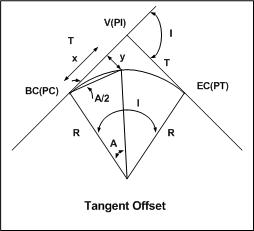* Log In to use the Calculate function * Become a Member!

Calculate The Interior Angle To Tangent Offset:

Calculation:
Designer/Checker:

Input:Radius of curve (R - ft or m)
Distance along tangent to point of offset (x - ft or m)

Output: